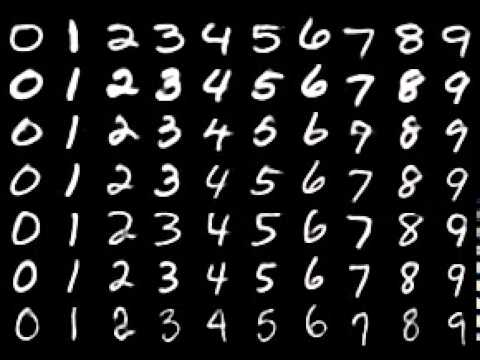NASA's Punk Music

# npm

## mnist

1.1.0 • Public • Published

# MNIST DigitsThe goal of this library is to provide an easy-to-use way for training and testing MNIST digits for neural networks (either in the browser or node.js). It includes 10000 different samples of mnist digits. I built this in order to work out of the box with Synaptic.

You are free to create any number (from 1 to 60 000) of different examples c via MNIST Digits data loader

### Installation

for node.js: `npm install mnist --save`

for the browser: `bower install mnist --save`

### Usage

The most important method is `mnist.set(trainingAmount, testAmount)` which takes the amount of samples for the training and test sets, and returns an object with the two sets of samples (one for training and the other one for testing). Both sets are shuffled, and there are no samples repeated in both sets.

For example:

``````var mnist = require('mnist'); // this line is not needed in the browser

var set = mnist.set(8000, 2000);

var trainingSet = set.training;
var testSet = set.test;

``````

That would create a random training set of 8000 mnist digits, and a test set with other random 2000 mnist digits, and there are not going to be any sample in the training set repeated in the test set.

Every set consist of an array of elements, which each of them will looks like this:

``````{
input: [0,0,0,1,1, ... ,0,0], // a 784-length array of floats representing each pixel of the 28 x 28 image, normalized between 0 and 1
output: [0,0,0,0,0,0,1,0,0,0] // a 10-length binary array that tells which digits (from 0 to 9) is in that image
}
``````

### Helpers

Every digit has a set of helper functions which are listed below. To access these functions you have to target the desired digit like this: `mnist[digit]`.

So, for example, to get a single '5' digit, you would call `mnist.get()`.

These are all the helper function:

##### .get([index])

Returns a single sample of that digit, given its index. If no index is provided, a random sample is returned.

``````mnist.get(100) // [0,0,0,1,1...,0,0]
``````
##### .length

Returns the number of samples available for that digit

``````mnist.length // 1009
``````
##### .range(start, end)

Returns an array of samples, corresponding to the range specified between the `start` and `end` arguments

``````mnist.range(10, 17) // [ array with 7 samples of the digit 9 ]
``````
##### .set(start, end)

Returns an dataset of samples, corresponding to the range specified between the `start` and `end` arguments, every element of the array has an `input` and `output` properties, being `input` the normalized data for all the pixels of each image, and output a 10-length binary array representing which digit is in that image.

``````mnist.set(200, 250) // [ dataset with 50 samples of the digit 4 ready for training/testing a neural network ]
``````
##### .raw

This property provides access to all the raw data for all the samples of that digit

``````mnist.raw // all the raw data for digit 3
``````

### Drawing a digit (only in the browser)

This lets you draw a given digit in a canvas context: `mnist.draw(digit, context [,offsetX, offsetY])`

``````var digit = mnist.get();
var context = document.getElementById('myCanvas').getContext('2d');

mnist.draw(digit, context); // draws a '1' mnist digit in the canvas
``````

The digit provided has to be a 784-length array of normalized values (0-1). All of the following approaches will work:

``````var digit = mnist.get(); // single digit
var digit = mnist.range(0, 100); // extracting the digit from a range
var digit = mnist.set(0, 100).input; // extracting the digit from a dataset
var digit = mnist.set(8000, 2000).training.input; // extracting the digit from a training set
var digit = mnist.set(8000, 2000).test.input; // extracting the digit from a test set
``````

### Contributing

If you want to contribute feel free to submit PR's, just make sure to run `npm run build` to build the `/dist` files before submitting.

## Keywords

### Install

`npm i mnist`

### Repository

github.com/cazala/mnist

### Homepage

github.com/cazala/mnist